Home | | Strength of Materials II | Strength of Materials- State of Stress in Three Dimensions

# Strength of Materials- State of Stress in Three Dimensions

Spherical and deviatory components of stress tensor- determination of principal of principal stresses and principal planes -volumetric strain- dilation and distortion -Theories of failure -principal stress dilatation. Principal strain -shear stress - strain energy and distortion energy theories - application in analysis of stress. Load carrying capacity and design of members -interaction problems and interaction curves -residual stresses.

STATE OF STRESS IN THREE DIMENSIONS

1 STRESS

When a certain system of external forces act on a body then the body offers resistance to  these forces. This internal resistance offered by the body per unit area is called the stress  induced in the body.

2 PRINCIPAL PLANES

The plane in which the shear stress is zero is called principal planes. The plane which is  independent of shear stress is known as principal plane.

3 SPHERICAL TENSOR4 DEVIATOR STRESS TENSOR5 THE STRESS COMPONENTS AT A POINT ARE GIVEN BY THE FOLLOWING ARRAY

Calculate the principal stress and principal planes.Solution:

The principal stresses are the roots of the cubic equation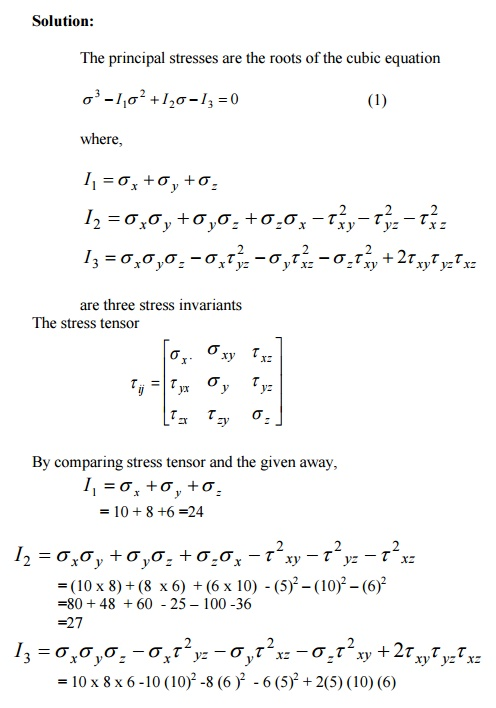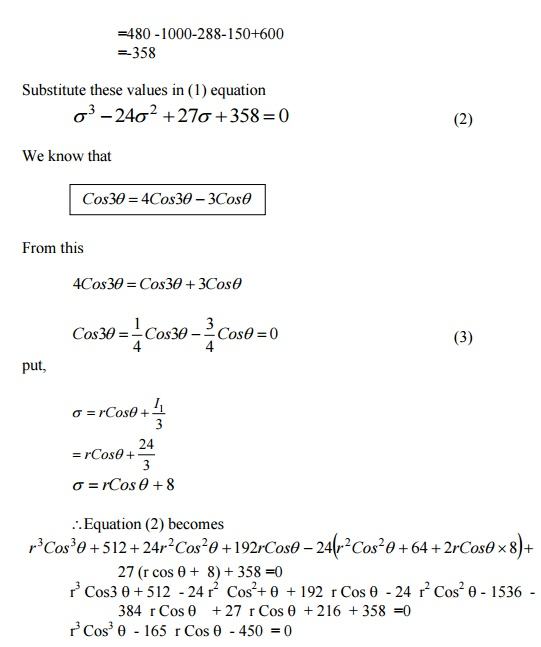6 OBTAIN THE PRINCIPAL STRESSES AND THE RELATED DIRECTION COSINES  FOR THE FOLLOWING STATE OF STRESS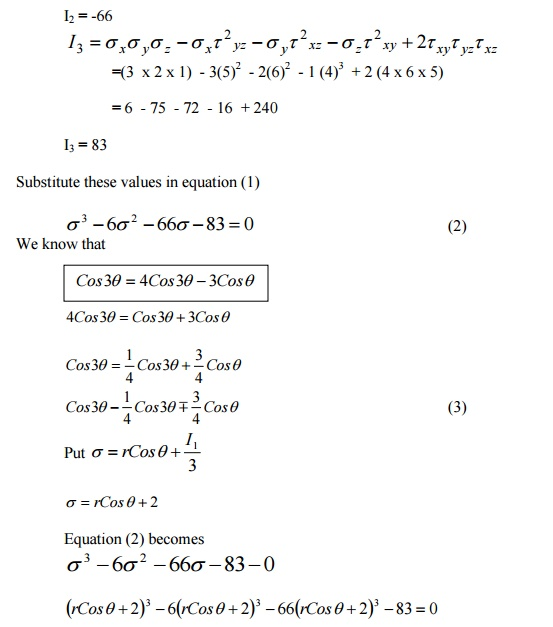THE STATE OF STRESS AT A POINT IS GIVEN BY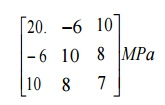Determine the principal stresses and principal direction.

Solution: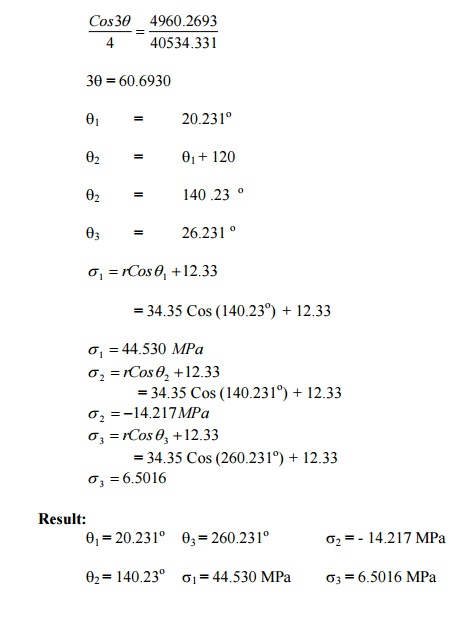9 EXPLAIN THE ENERGY OF DISTORTION ( SHEAR STRAIN ENERGY ) AND DILATATION

The strain energy can be split up on the following two strain energies.

i.                                                                                        Strain energy of distortion (shear strain energy)

ii.                                                                                      Strain energy of Dilatation (Strain energy of uniform compression (or)) tension  (or) volumetric strain energy )

Let e1 e2 an d e3  be the principal strain in the directions of principal stresses s1, s2 and  s3.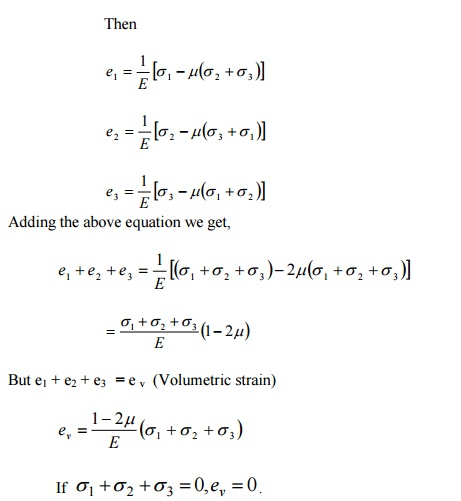.  This means that if sum of the three principal stress is  zero there is no volumetric change, but only the distortion occurs.

From the above discussion,

1.                 When the sum of three principal stresses is zero, there is no volumetric change but only the distortion occurs.

2.                                                                                      When the three principal stresses are equal to one another there is no distortion  but only volumetric change occurs.

Note:

In the above six theories,

set  , sec = Tensile stress at the elastic limit in simple tension and compression;

s1, s2, s3 =  Principal stresses in any complex  system  (such that e1 > e2 > e3 )

10 STATE THE PRINCIPAL THEORIES OF FAILURE

1.                  Maximum principal stress theory

2.                  Maximum shear stress (or) stress difference theory

3.                  Strain energy theory

4.                  Shear strain energy theory

5.                  Maximum principal strain theory

6.                 Mohr?s   Theory

11 LIMITATIONS OF MAXIMUM PRINCIPAL STRESS THEORY

1.                                                                    On  a  mild  steel  specimen  when  spiel  tension  test  is  carried  out  sliding  occurs  approximately 45o to the axis of the specimen; this shows that the failure in this case is  due to maximum shear stress rather than the direct tensile stress.

2.                                                                    It has been found that a material which is even though weak in simple compression yet  can sustain hydrostatic pressure for in excess of the elastic limit in simple compression.

12 MAXIMUM PRINCIPAL STRESS THEORY

According to this theory failure will occur when the maximum principle tensile stress  (s1) in the complex system reaches the value of the maximum stress at the elastic limit (set) in the simple tension.

13 MAXIMUM SHEAR STRESS THEORY

This theory implies that failure will occur when the maximum shear stress  in the complex system reaches the value of the maximum shear stress in simple tension at elastic limit (i.e)14 LIMITATIONS OF MAXIMUM SHEAR STRESS THEORY

i.                                                                            The theory does not give accurate results for the state of stress of pure shear in which  the maximum amount of shear is developed (i.e) Torsion test.

ii.                                                                          The theory does not give us close results as found by experiments on ductile  materials. However, it gives safe results.

15 SHEAR STRAIN ENERGY THEORY

According to this theory the elastic failure occurs where the shear strain energy per unit  volume in the stressed material reaches a value equal to the shear strain energy per unit volume  at the elastic limit point in the simple tension test.

16 LIMITATIONS OF DISTORTION ENERGY THEORY

1.                             The theory does to agree the experiment results for the material for which sat is  quite different etc.

2.                                                                          This theory is regarded as one to which conform most of the ductile material under  the action of various types of loading.

17   MAXIMUM PRINCIPAL STRAIN THEORY

The theory states that the failure of a material occurs when the principal tensile strain in  the material reaches the strain at the elastic limit in simple tension (or) when the min minimum  principal strain (ie ) maximum principal compressive strain reaches the elastic limit in simple compression.

18 LIMITATIONS IN MAXIMUM PRINCIPAL STRAIN THEORY

i.                                                                                        The theory overestimates the behaviour of ductile materials.

ii.                                                                                      The theory does no fit well with the experimental results except for brittle  materials for biaxial tension.

19 STRESS TENSOR IN CARTESIAN COMPONENTS20 THREE STRESS INVARIANTS

The principal stresses are the roots of the cubic equation,21 TWO TYPES OF STRAIN ENERGY

i.                                                                                                                Strain energy of distortion (shear strain energy)

ii.                                                                                                              Strain energy of dilatation.

MOHR'S                        THEORYTotal Strain Energy Theory

The total strain energy of deformation is given by22 EXPLAIN  THE   MAXIMUM   PRINCIPAL   STRESS   THEORY:

-                                                                                  This is the simplest and the oldest theory of failure

-                                                                                  According to this theory failure will occur when the maximum principle tensile  stress (?1) in the complex system reaches the value of the maximum stress at the  elastic limit (?et) in the simple tension (or) the minimum principal stress (that is, the  maximum principal compressive stress), reaches the elastic limit stress (?) in  simple compression.

(ie.) ?1?et  (in simple tension)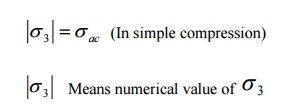-                                                                      If the maximum principal stress is the design criterion, the maximum principal stress  must not exceed the working sfor the material. Hence,

?1  ?

-                                                                      This theory disregards the effect of other principal stresses and of the shearing stresses  on other plane through the element. For brittle materials which do not fail by yielding  but fail by brittle fracture, the maximum principal stress theory is considered to be  reasonably satisfactory.

This theory appears to be approximately correct for ordinary cast -irons and brittle metals.

23 THE MAXIMUM PRINCIPAL STRESS THEORY IS CONTRADICTED IN THE  FOLLOWING CASES:

On a mild steel specimen when simple tension test is carried out sliding occurs approximately 45o to the axis of the specimen; this shows that the failure in the case is due to maximum shear stress rather than the direct tensile stress.

2.                                                                                      It has been found that a material which is even though weak in simple  compression yet can sustain hydrostatic pressure for in excess of the elastic limit  in simple compression.

24 EXPLAIN THE MAXIMUM SHEAR STRESS (OR) STRESS DIFFERENCE THEORY

- This theory implies that failure will occur when the maximum shear stress  maximum in the complex system reaches the value of the maximum shear  stress in simple tension at the elastic limit i.e.In actual design set  in the above equation is replaced by the safe stress.

-                                                                                This theory gives good correlation with results of experiments on ductile materials.  In the case of two dimensional tensile stress and then the maximum stress difference calculated to equate it to set.

Limitations of this theory:

i.                                                                                        The theory does not give accurate results for the state of stress of pure shear in  which the maximum amount of shear is developed (ie) Torsion test.

ii.                                                                                      The theory is not applicable in the case where the state of stress consists of  triaxial tensile stresses of nearly equal magnitude reducing, the shearing stress to  a small magnitude, so that failure would be by brittle facture rather than by yielding.

iii.                                                                                   The theory does not give as close results as found by experiments on ductile  materials. However, it gives safe results.

25 EXPLAIN THE SHEAR STRAIN ENERGY THEORY

This theory is also called 'Distortion-Henky E Theory'

- According to this theory the elastic failure occurs where the shear strain energy per  unit volume in the stressed material reaches a value equal to the shear strain  energy per unit volume at the elastic limit point in the simple tension test.

Shear strain energy due to the principal stresses s1, s2, and s3 per unit volume of the  stress material.But for the simple tension test at the elastic limit point where there is only one principal  stress (ie) set we have the shear strain energy per unit volume which is given byThe above theory has been found to give best results for ductile material for which set =sec approximately.

Limitations of Distortion energy theory:

1.                                                                          Te theory does to agree with the experimental results for the material for which ?et is  quite different from ?ec.

2.                                                                          The theory gives ?et =0 for hydrostatic pressure (or) tension, which means that the  material will never fail under any hydrostatic pressure (or) tension. When three equal  tensions are applied in three principal directions, brittle facture occurs and as such  maximum principal stress will give reliable results in this case.

3.                                                                          This theory is regarded as one to which conform most of the ductile material under  the action of various types of loading.

26 EXPLAIN THE MAXIMUM PRINCIPAL STRAIN THEORY

-                                                                                        This theory associated with St  Venent

-                                                                                        The theory states that the failure of a material occurs when the principal tensile  strain in the material reaches the strain at the elastic limit in simple tension (or)  when the minimum principal strain (ie) maximum principal compressive strain  reaches the elastic limit in simple compression.

Principal strain in the direction of principal stress ?1,Limitations:

i.                                                                The theory overestimates the behavior of ductile materials.

ii.                                                              Te theory does not fit well with the experimental results except for brittle materials for  biaxial tension.

27 EXPLAIN THE STRAIN ENERGY THEORY

The total stain energy of deformation is given byThe total elastic energy stored in a material before it reaches the plastic state can have no  significance as a limiting condition, since under high hydrostatic pressure, large amount of strain  energy ma be stored without causing either fracture (or) permanent deformation.

28 EXPLAIN  MOHR'S   THEORY

A material may fail either through plastic slip (or) by fracture when either the shearing  stress in the planes of slip has increased.This equation represents the family of major principal stress circles in parameter form. The equation of this envelope is obtained by partially differentiating with respect to PThis is to equation of Mohr? principal stress in parameter form.

IN A STEEL MEMBER, AT A POINT THE MAJOR PRINCIPAL STRESS IS 180  MN/M2 AND THE MINOR PRINCIPAL STRESSES IS COMPRESSIVE. IF THE  TENSILE YIELD POINT OF THE STEEL IS 225 MN/M2, FIND THE VALUE OF  THE MINOR PRINCIPAL STRESS AT WHICH YIELDING WILL  COMMENCE, ACCORDING TO EACH OF THE FOLLOWING CRITERIA OF FAILURE.

i.                                                                                                                Maximum shearing stress

ii.                                                                                                              Maximum total strain energy

iii.                                                                                                           Maximum shear strain energy

Take Poisson's   ratio   =   0.26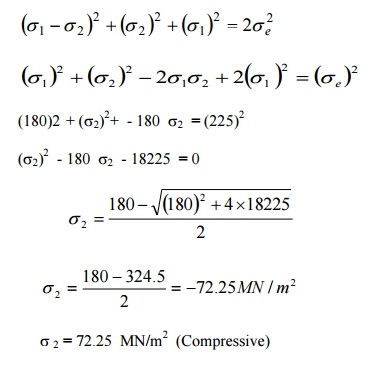IN A MATERIAL THE PRINCIPAL STRESSES ARE 60 MN/M2, 48 MN/M2 AND - 36  MN/M2. CALCULATE

i.                                                                                                                            TOTAL STRAIN ENERGY

ii.                                                                                                                          VOLUMETRIC STRAIN ENERGY

iii.                                                                                                                       SHEAR STRAIN ENERGY

iv.                                                                                                                       FACTOR OF SAFETY ON THE TOTAL STRAIN ENERGY  CRITERIA IF THE MATERIAL YIELDS AT 120 MN/M2.

TAKE E = 200 GN/M2+ AND 1/M = 0.3IN A MATERIAL THE PRINCIPAL STRESSES ARE 50 N/MM2, 40 N/MM2 AND - 30 N/MM2, CALCULATE:

i.                                                                                                               TOTAL STRAIN ENERGY

ii.                                                                                                             VOLUMETRIC STRAIN ENERGY

iii.                                                                                                           SHEAR STRAIN ENERGY AND

iv.                                                                                                           FACTOR OF SAFETY ON THE TOTAL STRAIN ENERGY  CRITERION IF THE MATERIAL YIELD AT 100 N/MM2.

iii.                                                                                                           TAKE E = 200 X 103 N/MM2 AND POISSION RATIO = 0 .28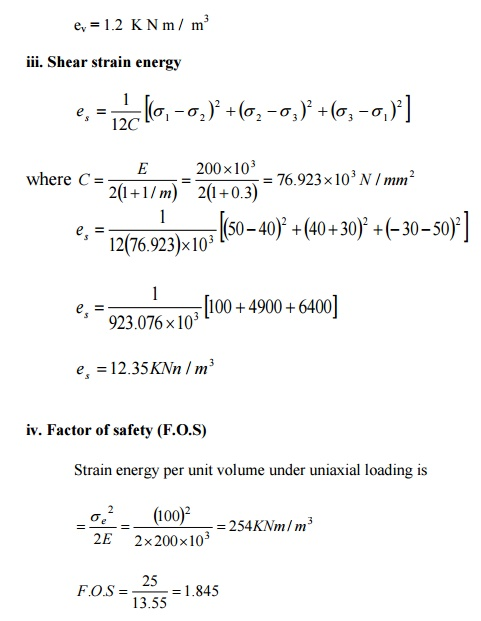IN A MATERIAL THE PRINCIPAL STRESSES ARE 50 N/MM2, 40 N/MM2 AND - 30  N/MM2, CALCULATE:

v.                                                                                                              TOTAL STRAIN ENERGY

vi.                                                                                                           VOLUMETRIC STRAIN ENERGY

vii.                                                                                                         SHEAR STRAIN ENERGY AND

viii.                                                                                                      FACTOR OF SAFETY ON THE TOTAL STRAIN ENERGY  CRITERION IF THE MATERIAL YIELD AT 100 N/MM2.

TAKE E = 200 X 103 N/MM2 AND POISSION RATIO = 0 .2829 SHEAR STRAIN ENERGY PER UNIT VOLUME30 THEORIES OF FAILURE

The principal theories are:

1.       Maximum principal stress theory

2.       Maximum shear stress (or) stress difference theory

3.       Strain energy theory

4.       Shear strain energy theory

5.       Maximum principal strain theory

Study Material, Lecturing Notes, Assignment, Reference, Wiki description explanation, brief detail
Civil : Strength of Materials : State of Stress in Three Dimensions : Strength of Materials- State of Stress in Three Dimensions |